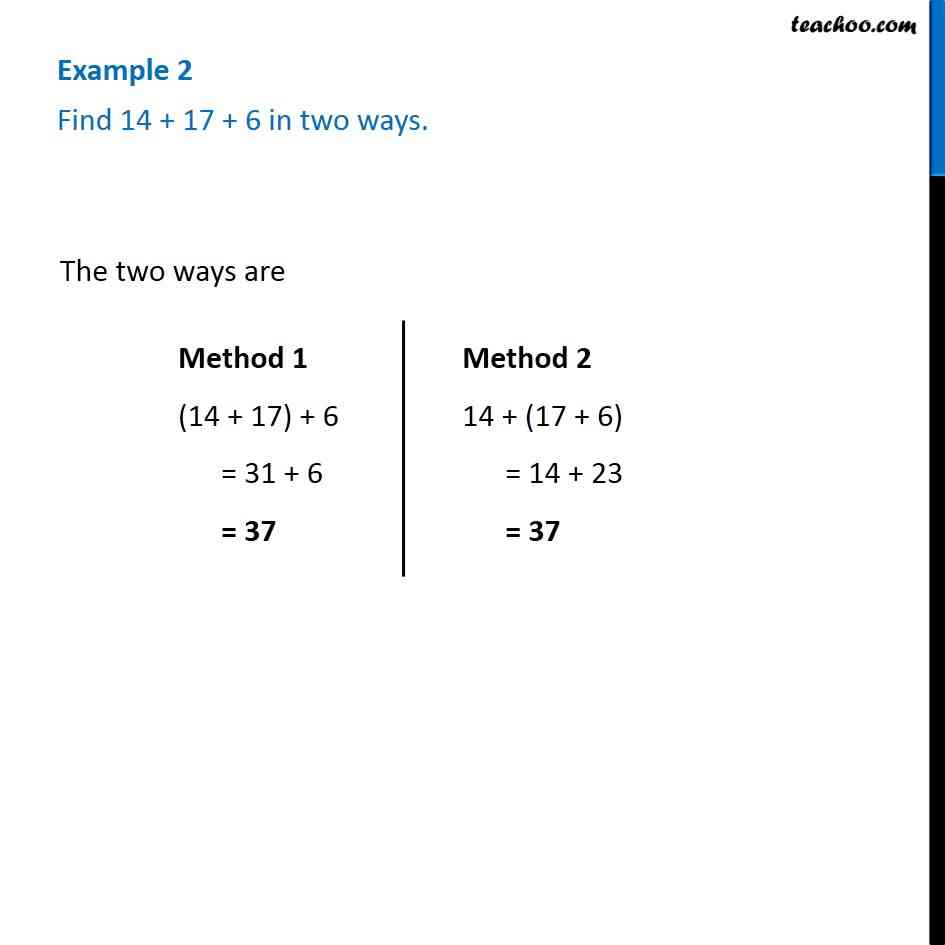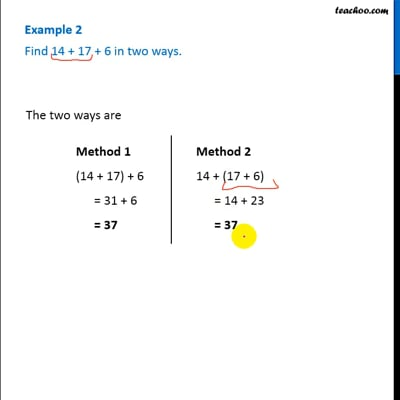Multiplication and Addition of Whole numbers

Chapter 2 Class 6 Whole Numbers
Concept wiseThis video is only available for Teachoo black users

### Transcript

Example 2 - Chapter 2 Class 6 Whole Numbers - NCERT Book Find 14 + 17 + 6 in two ways. The two ways are Method 1 (14 + 17) + 6 = 31 + 6 = 37 Method 2 14 + (17 + 6) = 14 + 23 = 37